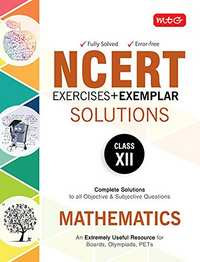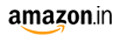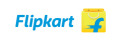Share

# NCERT Exercises + Exemplar Solutions Mathematics - Class 12 CBSE (Science) Class 12

SubjectNCERT Exercises + Exemplar Solutions Mathematics - Class 12By MTG Editorial Board (Author)
M.R.P 250/-170.00(32%)319.00-
Product Description
##### lok

• Relations and Functions
• Inverse Trigonometric Functions
• Matrices
• Determinants
• Continuity and Differentiability
• Application of Derivatives
• Integrals
• Application of Integrals
• Differential Equations
• Vector Algebra
• Three Dimensional Geometry
• Linear Programming
• Probability

S